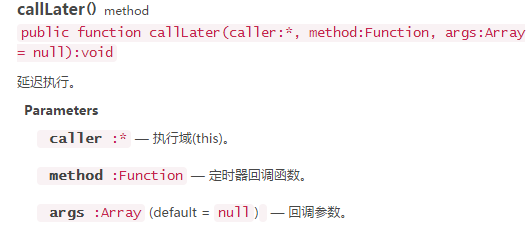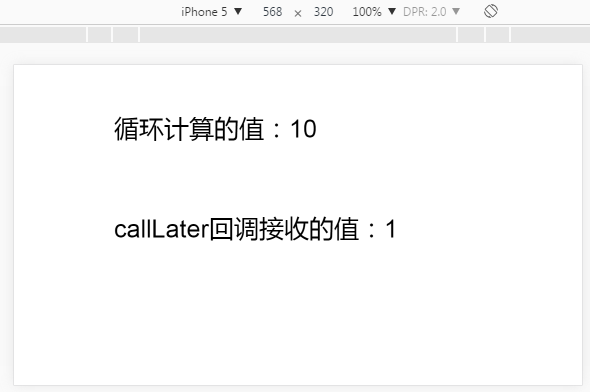# LayaAir引擎计时器：延迟调用API（JS）

711501594

延迟调用callLater()是时钟管理类laya.utils.Timer中的一个常用方法。callLater是在本帧渲染之前，延迟调用某个回调函数，同一个回调函数是排重的，只会被调用一次。在涉及重复调用某个函数，使用延迟调用方法可避免重复运算或重复渲染，从而达到性能优化的目的。我们先看下该方法的API参数说明，如下图所示：callLater示例代码：

 123456789101112131415161718192021222324252627282930313233343536 `(``function``()``{``    ``var` `Text     = Laya.Text;``    ``var` `WebGL   = Laya.WebGL;` `    ``(``function``()``    ``{``        ``Laya.init(1136, 640, WebGL);``        ` `        ``Laya.stage.bgColor = ``"#ffffff"``;``        ` `        ``var` `s=0;``        ``for` `(``var` `i = 1; i < 5; i++)``        ``{``            ``s += i;``            ``//尽管处于循环中，callLater中的回调在渲染时仅执行一次，不会重复调用``            ``Laya.timer.callLater(``this``, onCallLater, [s]);``        ``}``        ``//显示for循环计算的值``        ``showText(``"循环计算的值："``, s, 200, 100);          ``        ` `    ``})();` `    ``function` `onCallLater(s)``    ``{``        ``showText(``"callLater回调接收的值："``, s, 200, 300);``    ``}``    ``function` `showText(t,i,x,y)``    ``{``        ``var` `text = ``new` `Text();``        ``text.text = t + i; ``        ``text.fontSize = 50;``        ``text.pos(x,y);                                ``        ``Laya.stage.addChild(text);   ``    ``}``})();`在本次示例，for循环中采用callLater延迟执行，从运行结果上看，回调函数中显示接收的值是第一次调用的值。而循环外获得了完整的计算结果。充分说明在callLater中的回调方法，尽管被循环调用了多次，但都会被放到延迟队列中，在渲染时执行最早的调用。